No items found.Written byJune 26, 2020

#### Share & Star us:

Thank you! Your submission has been received!
Oops! Something went wrong while submitting the form.
More Blogs
Edited: First published

# Unifying Single-host and Distributed Machine Learning with Maggy

This blog covers the oblivious training function and the internals of Maggy presented at Spark+AI Summit 2020, on June 26th.

TLDR; Maggy is an open-source framework for distributed machine learning. In this post, we introduce a new unified framework for writing core ML training logic as “oblivious training functions”. Maggy enables you to reuse the same training code whether training small models on your laptop or reusing the same code to scale out hyperparameter tuning or distributed deep learning on a cluster. Maggy enables the replacement of the current waterfall development process for distributed ML applications, where code is rewritten at every stage, with an iterative development process.

Most of the publicly available ML source code for training models is not built to scale-out on many servers or GPUs. Getting started with deep learning is relatively easy these days, thanks to fast.ai, GitHub, and the blogosphere. The hard part for practitioners starts when the code examples found online need to be applied to more challenging domains, with larger and custom datasets, which in turn will require a bigger customized version of the model to fit that dataset. Using publicly available code as a starting point for model development on clusters, you will end up in a process similar to the one depicted in Figure 1.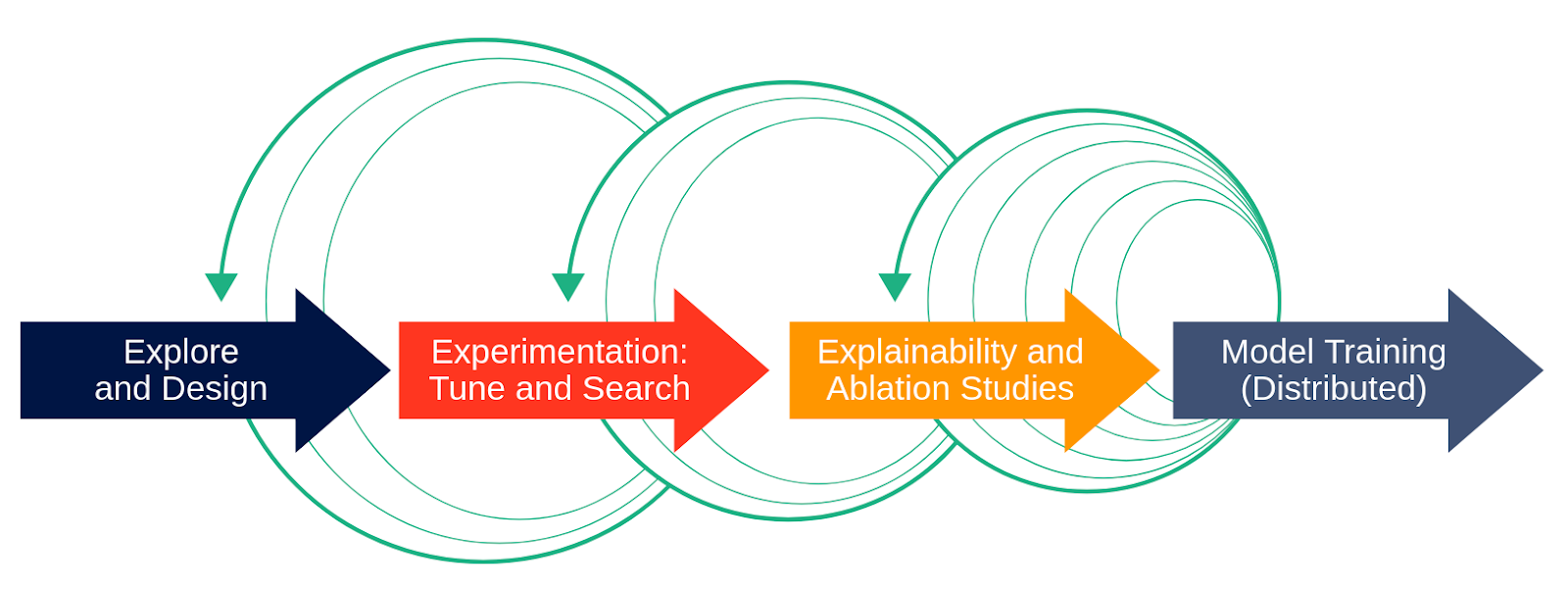Figure 1: A simplified view of the ML model development process, illustrating its iterative nature.

The software development process for ML models is rarely the perfect waterfall development model, as shown in Figure 1 without the green arrows. In the (discredited) waterfall development process, you would start out with requirements, then move on to design, implementation and test. The (current!) equivalent process in ML model development is the following, as shown in Figure 1 with the green arrows. You start out on your local machine with a subset of the data in order to explore and design the model architecture. Then you move to use a cluster of resources (such as GPUs) to more quickly find hyperparameters, run lots of parallel ablation studies (many skip this stage!), and finally scale out the training of the model on the large dataset using lots of resources. Then, you’re done, right? Wrong! You typically iterate through the stages, finding better hyperparameters, adding new features, rewriting for distribution, going from your laptop to the cluster and back again.

We rewrite our model training code for distribution as it offers many benefits – faster training of models using more GPUs, parallelizing hyperparameter tuning over many GPUs, and parallelizing ablation studies to help understand the behaviour and performance of deep neural networks. However, not only will the boiler plate model training code need to be modified, but as you move along the process, distribution will introduce additional obtrusive code artifacts and modifications, depending on the frameworks used. This will lead to a mix of infrastructure code and model code, with duplicated training logic, hyperparameters hard-coded into the training loop, additional tracking code to keep record of your changes and config files for experiments: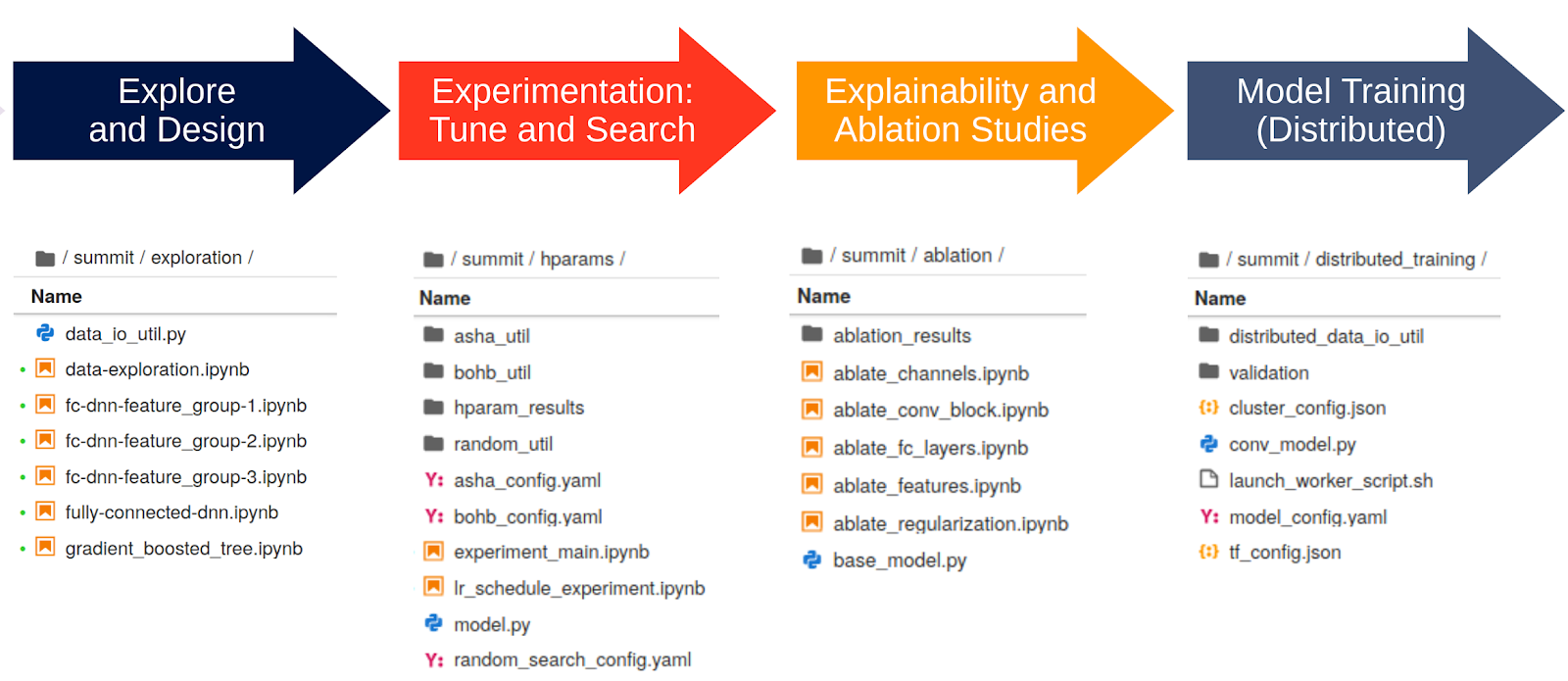Figure 2: Model development creates a mix of code artefacts duplicating code for every step, making iterative development hard.

With such a code base, iterating becomes near impossible as it requires adapting many copies of redundant code. And finally, imagine handing the code off to an ML engineer to productionize the model.

## The Oblivious Training Function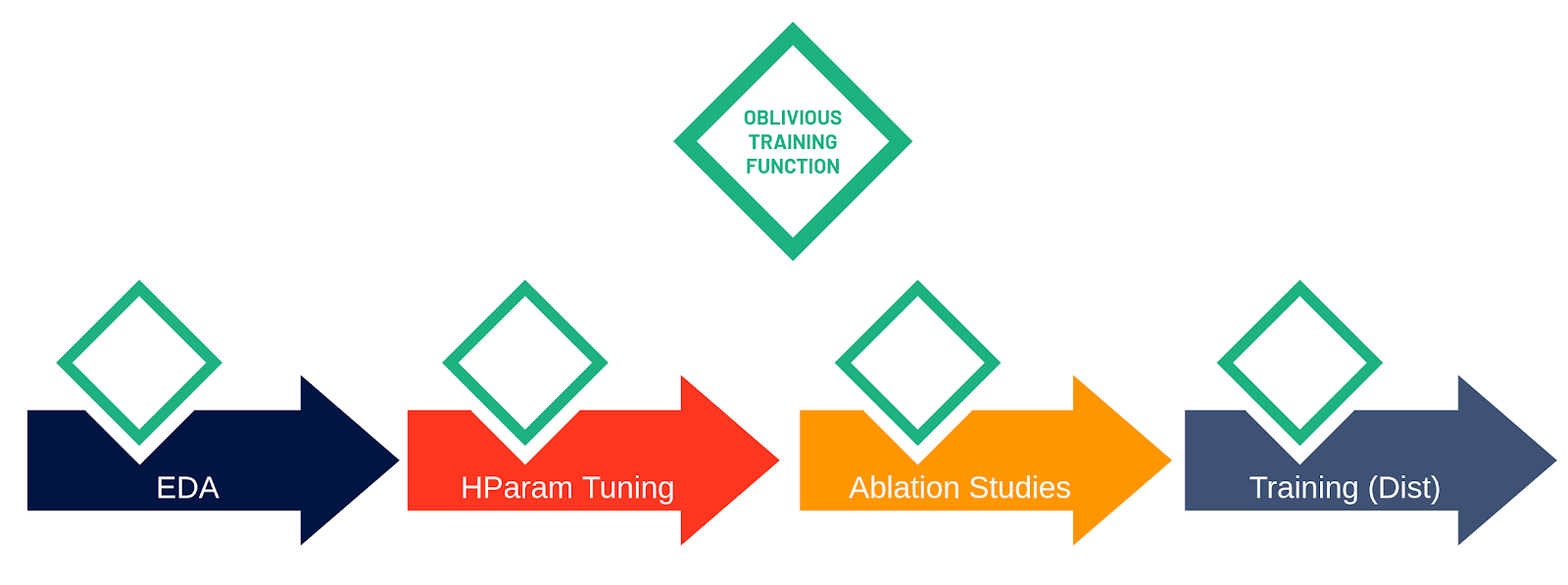Figure 3: The oblivious training function makes training code reusable among all steps of the process.

We introduce an open-source framework, Maggy, that enables write-once training functions that can be reused in single-host Python programs and cluster-scale PySpark or Distributed TensorFlow programs. Training functions written with Maggy look like best-practice TensorFlow programs where we factor out dependencies using popular programming idioms (such as functions to generate models and data batches). We call this new abstraction for ML model development the oblivious training function, as the core model training logic supports distribution transparency, that is, the training code is not aware (oblivious) of whether it is being run on a single host or whether it is being executed on hundreds of devices in parallel.

## What does it mean for training code to be distribution transparent?

Transparency in distributed systems refers to hiding distribution-specific aspects of an application from the developer - for example, a developer invoking a function may not know (or need to know) if the function she is calling is local to her application or on a remote server. This means, distribution transparency enables developers to write code that is reusable between single-host and distributed instantiations of a program: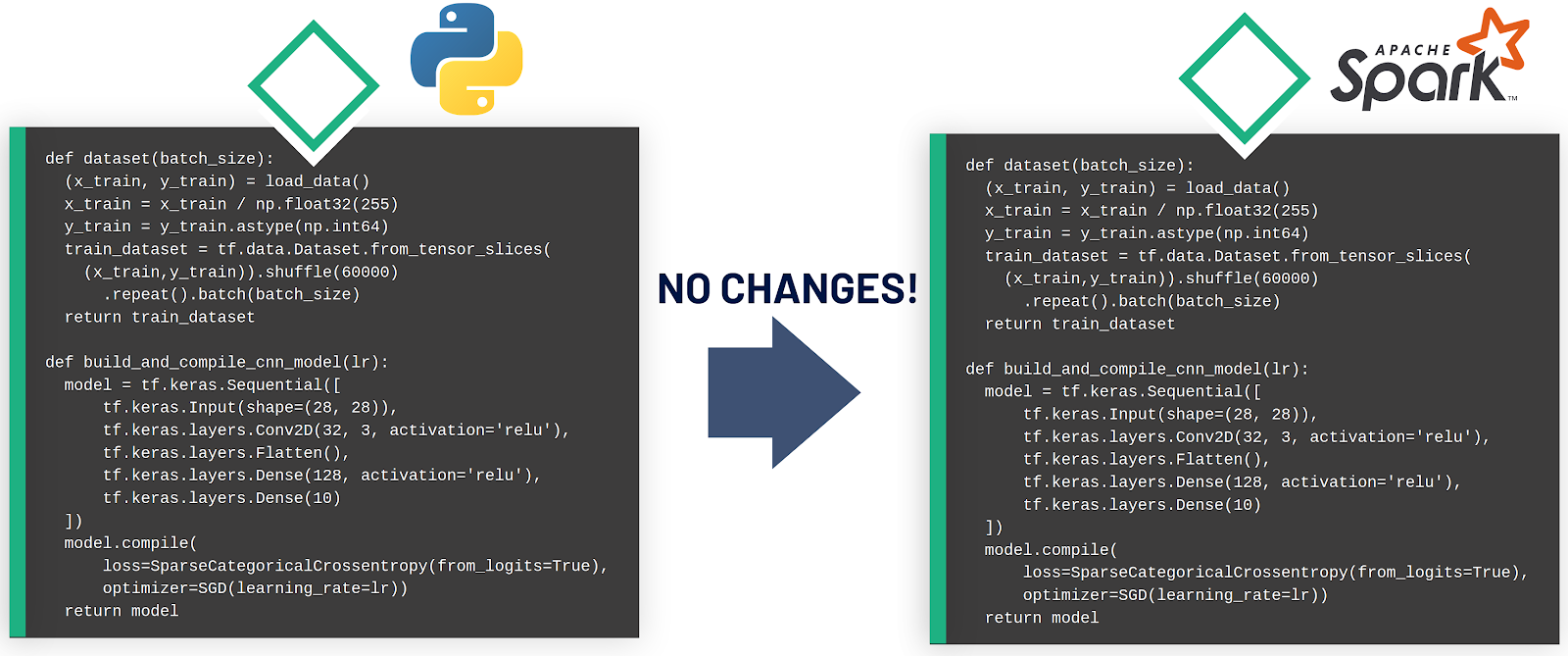Figure 4: Distribution Transparency hides complexities related to distribution from the developer, making the same code executable on a single-host as well as in a large cluster. Transparency leads to DRY training code.

## Building Blocks for Distribution Transparency

How does ML code have to be structured in order to be transparently distributed? Firstly, developers have to follow best practices and, secondly, developers must be aware of the difference between distribution contexts, that is, what characterizes, for example, distributed hyperparameter tuning vs. distributed training.

### 1. ML Development Best Practices:‍

The ML community has recently developed some best practices, which are already widely spread among developers. Taking a look at the new well-illustrated Keras Guides, you will notice a common approach with four techniques.

• Modularize: By modularizing code into reusable functions, these functions become building blocks, making the code pluggable in order to construct different configurations of the model for hyperparameter optimization or ablation.
• Parametrize: Instead of hardcoding parameters such as learning rate, regularization penalty or other hyperparameters, developers are encouraged to replace this with variables whenever possible, to have a single place for them to be changed.
• Higher order training functions: instead of using instantiated objects for example for the training dataset, the input logic related to the data can be encapsulated in a function which is being used by a higher order function. By doing so also the data input pipeline can be parametrized. The same holds for the generation of the model, which can be encapsulated in a function returning the model.
• Usage of callbacks at runtime: In order to be able to intercept and interact with the actual training loop, most ML frameworks such as TensorFlow and PyTorch offer the possibility to use callback functions that are  invoked by the framework at certain points in time during training, such as at the end of every epoch or batch. Callback functions  enable  runtime monitoring of training, and can, for example, also be used to add support to stop the training early (important in hyperparameter optimization).

### 2. Distribution Context‍

While a single-host environment is self-explanatory, there is a difference between the context of ML experiments, such as hyperparameter optimization or parallel ablation studies, and the distributed training of a single model. Both hyperparameter optimization and parallel ablation studies have weak scaling requirements (also known as embarrassingly parallel), because all workers execute independent pieces of work and have limited communication. For example, hyperparameter tuning involves training independent copies of the model with different hyperparameters or different architectures, in order to find the best performing configuration. Distributed training, however, is strong scaling, as it introduces  significant communication and coordination between the workers. As workers are training a single model, they continually exchange gradients, which are computed on independent shards of data (data parallel training). Many distributed training problems, in fact, become (network or disk) I/O bound as they scale.  Figure 5 illustrates the three contexts and the step in the model development process that they are applicable to.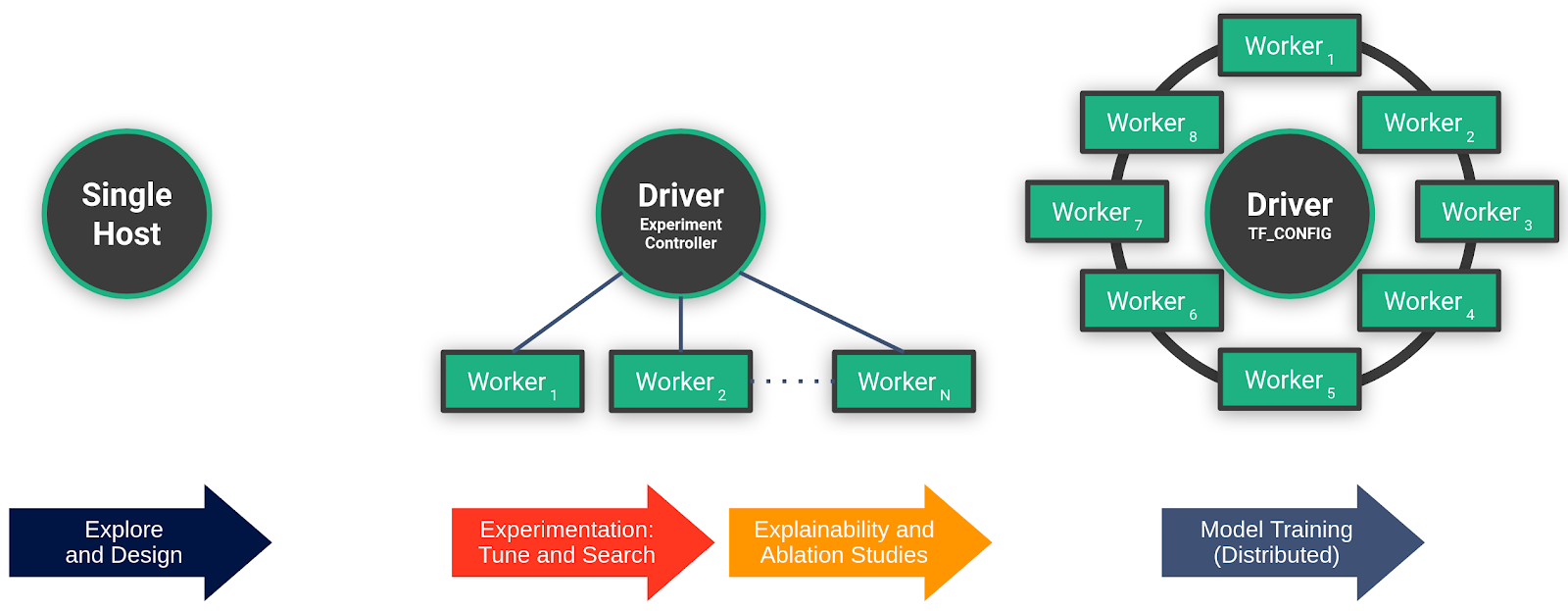Figure 5: Single-host vs. parallel multi-host vs. distributed multi-host context and their applicability to the steps of the process.

Being aware of the different contexts and applying popular programming idioms, it becomes apparent what it means for the oblivious training function. It is no longer the developer herself who instantiates and launches the training function, but the framework that will invoke the training function as it is aware of the current context and it will take care of the distribution related complexities. That means, for exploration, the framework can be used to fix all parameters. For hyperparameter optimization experiments, the framework will take care of generating potentially good hyperparameter combinations and parameterizing the oblivious training function with them to be launched on different workers. For distributed training, it means setting up the environment for workers to discover each other and wrapping the model code with a distribution strategy.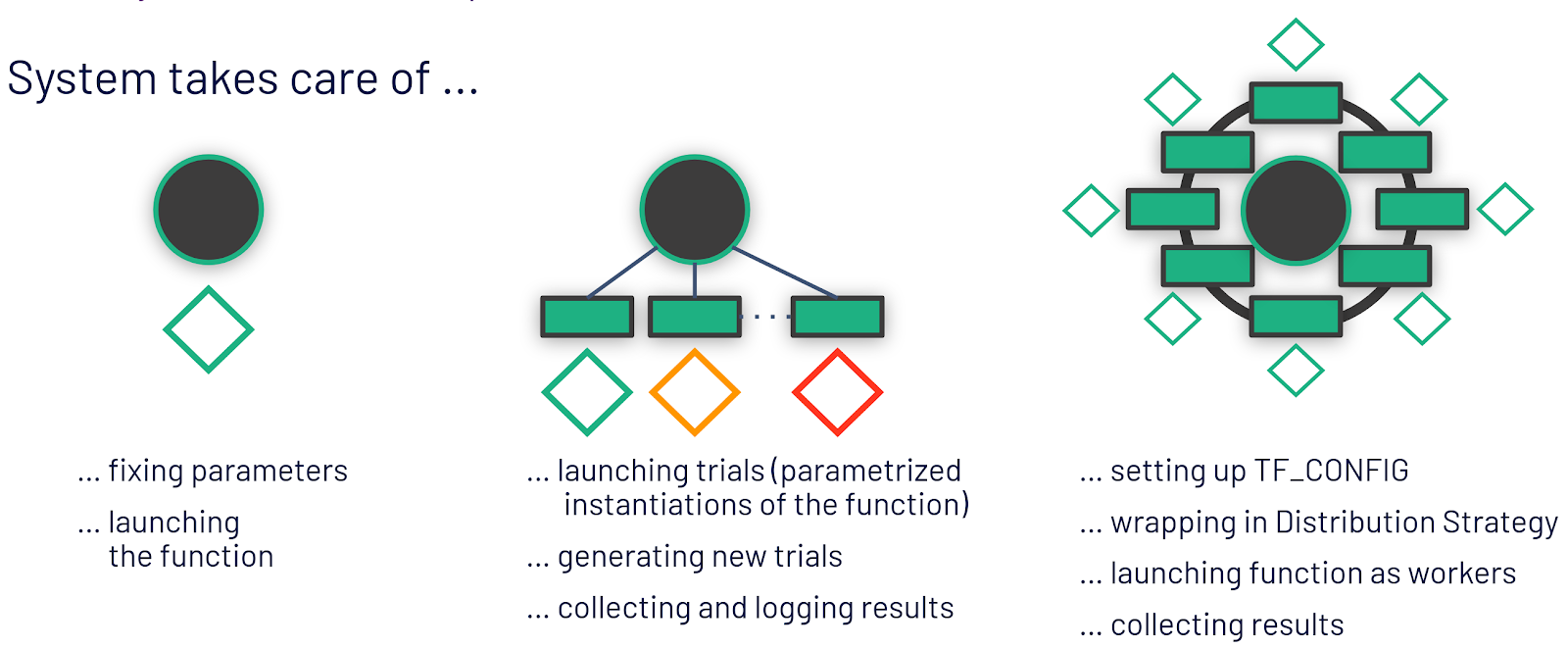Figure 6: The oblivious training function as an abstraction allows us to let the system take care of distributed system related complexities.

## ‍Putting it all together

Having the building blocks at hand, how do we write the model training code in Maggy? Let us take a look at the latest best-practices MNIST example that already factors the model configuration, dataset preparation and training logic into functions. Building on this example, we will show the modifications to the code that are needed to construct an oblivious training function in Maggy. It is important to note that all modifications are still vanilla Python code, and can, therefore, be run as is on a single host environment. Let’s start with the boiler plate with the two functions and the training logic:

### 1. Model Definition

def get_model():
model = tf.keras.Sequential([
tf.keras.Input(shape=(784,)),
tf.keras.layers.Reshape(target_shape=(28, 28, 1)),
tf.keras.layers.Conv2D(32, name='conv1', kernel_size=3, activation='relu'),
tf.keras.layers.MaxPooling2D(pool_size=2),
tf.keras.layers.Conv2D(64, name='conv2', kernel_size=2, activation='relu'),
tf.keras.layers.MaxPooling2D(name='pool1', pool_size=2),
tf.keras.layers.Flatten(),
tf.keras.layers.Dropout(0.1),
tf.keras.layers.Dense(128, activation='relu'),
tf.keras.layers.Dense(10)
])
return model

### 2. Data set generation

def get_dataset():
batch_size = 32
num_val_samples = 10000    # Return the MNIST dataset in the form of a `tf.data.Dataset`.
(x_train, y_train), (x_test, y_test) = tf.keras.datasets.mnist.load_data()    # Preprocess the data (these are Numpy arrays)
x_train = x_train.reshape(-1, 784).astype("float32") / 255
x_test = x_test.reshape(-1, 784).astype("float32") / 255
y_train = y_train.astype("float32")
y_test = y_test.astype("float32")    # Reserve num_val_samples samples for validation
x_val = x_train[-num_val_samples:]
y_val = y_train[-num_val_samples:]
x_train = x_train[:-num_val_samples]
y_train = y_train[:-num_val_samples]
return (
tf.data.Dataset.from_tensor_slices((x_train, y_train))
.shuffle(50000).repeat().batch(batch_size),
tf.data.Dataset.from_tensor_slices((x_val, y_val)).
.shuffle(10000).repeat().batch(batch_size),
tf.data.Dataset.from_tensor_slices((x_test, y_test))
.shuffle(10000).repeat().batch(batch_size),
)

### 3. Training logic

model = get_model()
model.compile(
loss=tf.keras.losses.SparseCategoricalCrossentropy(from_logits=True),
metrics=['accuracy', tf.keras.metrics.SparseCategoricalAccuracy()],
)# Train the model on all available devices.
train_dataset, val_dataset, test_dataset = get_dataset()
model.fit(train_dataset, epochs=2, steps_per_epoch=1000, validation_data=val_dataset, validation_steps=100)# Test the model on all available devices.
metrics = model.evaluate(test_dataset, steps=100)
metric_dict = {out: metrics[i] for i, out in enumerate(model.metrics_names)}

### 1. Model generation

We are parametrizing the model itself, by replacing hyperparameters with arguments.

Parametrizing the model definion

### 2. Dataset generation

The dataset generation function stays unchanged in this case, but similar to the model, this function could be parametrized

def get_dataset():
batch_size = 32
num_val_samples = 10000    # Return the MNIST dataset in the form of a `tf.data.Dataset`.
(x_train, y_train), (x_test, y_test) = tf.keras.datasets.mnist.load_data()    # Preprocess the data (these are Numpy arrays)
x_train = x_train.reshape(-1, 784).astype("float32") / 255
x_test = x_test.reshape(-1, 784).astype("float32") / 255
y_train = y_train.astype("float32")
y_test = y_test.astype("float32")    # Reserve num_val_samples samples for validation
x_val = x_train[-num_val_samples:]
y_val = y_train[-num_val_samples:]
x_train = x_train[:-num_val_samples]
y_train = y_train[:-num_val_samples]
return (
tf.data.Dataset.from_tensor_slices((x_train, y_train))
.shuffle(50000).repeat().batch(batch_size),
tf.data.Dataset.from_tensor_slices((x_val, y_val)).
.shuffle(10000).repeat().batch(batch_size),
tf.data.Dataset.from_tensor_slices((x_test, y_test))
.shuffle(10000).repeat().batch(batch_size),
)

### 3. Training logic

The training logic is wrapped in a parametrized and pluggable function, the oblivious training function. Again, hyperparameters are passed as arguments to the function. Additionally, the dataset and model generation functions are replaced with arguments, in order to be able to let the system, for example, replace the dataset generator with an alternative one - we use this to drop features for ablation studies. Last, but not least, the training function should return its current performance as a metric to be optimized in hyperparameter optimization. This is needed to make Maggy aware of the desired optimization metric.

Adjust Training Logic to be callable with different parameters

Note that up to this point, all modifications are pure Python code and, hence, the training function can still be run in a single host environment by calling it yourself in a Notebook with a fixed set of parameters and by passing the model and dataset generation functions as arguments.

Finally, to execute the function in a different distribution context, Maggy is used:

from maggy import experiment
experiment.set_dataset_generator(gen_dataset)
experiment.set_model_generator(gen_model)# Hyperparameter optimization
experiment.set_context('optimization', 'randomsearch', searchspace)
result = experiment.lagom(train_fun)
params = result.get('best_hp')# Distributed Training
experiment.set_context('dist_training', 'MultiWorkerMirroredStrategy', params)
experiment.lagom(train_fun)# Ablation study
experiment.set_context('ablation', 'loco', ablation_study, params)
experiment.lagom(train_fun)

Maggy requires additional configuration information for hyperparameter optimization, such as a search space definition and the optimization strategy to be used. In the case of distributed training, the distribution strategy is needed as well as a set of parameters to fix the model to. These parameters can either be taken from the previous hyperparameter tuning experiments or input manually. Lagom is the API to launch the function on a Spark cluster.

## Future Work

You can try out Maggy for hyperparameter optimization or ablation studies now on Hopsworks.ai and keep an eye on Maggy's GitHub repo for the oblivious training function to be released as a pure Spark version or wait until the next release of Hopsworks, that will include full support. Maggy is still a project under heavy development and our mission with Maggy is to provide a new way of writing machine learning applications that reduces the burden on Data Scientists becoming distributed systems experts. By following the best practices we are able to keep the high-level APIs of frameworks like Keras and PyTorch free of distribution obtrusive code.

## Summary

In this blog, we introduced a new feature to an open-source framework, Maggy, that enables write-once training functions that can be reused in single-host Python programs and cluster-scale PySpark programs. Training functions written with Maggy look like best-practice TensorFlow programs where we factor out dependencies using popular programming idioms (such as functions to generate models and data batches). In a single Jupyter notebook, developers can mix vanilla Python code to develop and test models on their laptop with PySpark-specific cells that can be run when a cluster is available using a PySpark kernel, such as Sparkmagic. This way, iterative development of deep learning models now becomes possible, moving from the laptop to the cluster and back again, with DRY code in the training function – as all phases reuse the same training code.

## References

Meister et al. (2020). Towards Distribution Transparency for Supervised ML With Oblivious Training Functions. Published in the MLOps workshop of MLSys’20.

Hello Asynchronous Search for PySpark, published on October 19, 2019.